+

# Asset Management Ratios

Author: Sophia Tutorial
##### Description:

Analyze asset management ratios.

(more)### Developing Effective Teams

*No strings attached. This college course is 100% free and is worth 1 semester credit.

37 Sophia partners guarantee credit transfer.

299 Institutions have accepted or given pre-approval for credit transfer.

* The American Council on Education's College Credit Recommendation Service (ACE Credit®) has evaluated and recommended college credit for 32 of Sophia’s online courses. Many different colleges and universities consider ACE CREDIT recommendations in determining the applicability to their course and degree programs.

Tutorial

what's covered
In this lesson, you will learn about asset management ratios and what they indicate about a business's operations. Specifically, this lesson will cover:
1. Asset Management Ratios
1. Inventory Turnover Ratio
2. Day Sales Outstanding Ratio
3. Fixed Assets Turnover Ratio
4. Total Assets Turnover Ratio

## 1. Asset Management Ratios

Asset management ratios are important to the area of financial statement analysis because they give an indication of how well assets are being managed internally in an organization and externally as compared to competitive companies or elsewhere in the industry.

Asset management ratios include:

• Inventory turnover ratio
• Days sales outstanding ratio
• Fixed asset turnover ratio
• Total assets turnover ratio
1a. Inventory Turnover Ratio
Inventory turnover ratio is a ratio that measures the number of times inventory is sold or used in a time period. It is calculated by dividing the cost of goods sold by the average inventory. The average inventory is calculated by taking the beginning and ending inventory for the balance sheet period and dividing that in half. This provides an estimate of the balance for the inventory at any point during the period.

formula
Inventory Turnover Ratio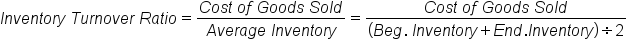### IN CONTEXT

XYZ Company has a cost of goods sold of \$750,000 for the year and an average inventory of \$300,000. What is the inventory turnover?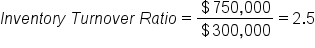When we divide the cost of goods sold by the average inventory, we get 2.5. This ratio is expressed as “2.5 times” meaning XYZ Company has turned over its inventory 2.5 times.

A low turnover rate may point to overstocking, obsolescence, or deficiencies in the product line or in the marketing effort. However, in some instances, a low rate may be appropriate, such as when higher inventory levels occur in anticipation of prices rising or expected market shortages.

Conversely, a high turnover rate may indicate inadequate inventory levels, which could lead to a loss in business because inventory is too low. This can often result in stock shortages.

hint
An item whose inventory is sold once a year has a higher holding cost than an item whose inventory is sold two or three times a year.

Inventory turnover also indicates the briskness of a business. The purpose of increasing inventory turnover is to reduce inventory for the following reasons:

• Increasing inventory reduces holding costs
• Reducing holding costs increases net income and profitability
• Items that turnover more quickly increase responsiveness to changes in customer requirements and allow the replacement of obsolete items
hint
The cost of goods sold can be found on the income statement and the average inventory can be found on the balance sheet.

terms to know

Inventory Turnover Ratio
A ratio that measures the number of times inventory is sold or used in a time period.
Holding Cost
Money spent to keep and maintain a stock of goods in storage.
1b. Day Sales Outstanding Ratio
The day sales outstanding ratio measures the company’s average collection period. It is a measure of their accounts receivable and how long it takes a company to collect the money for purchases that have been made. This ratio is considered an important tool in managing liquidity. Day sales outstanding tends to increase as a company becomes less risk averse, and a higher day sales outstanding can also be an indication of inadequate analysis of credit applicants.

The day sales outstanding ratio can be calculated by dividing the accounts receivable by the average day sales. The average day sales is the total sales for the year divided by 365.

formula

Day Sales Outstanding Ratio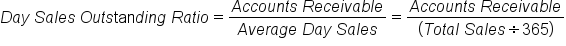hint
In this calculation, 365 days is used, rather than 360.

### IN CONTEXT

LMN Company has an accounts receivable of \$9,750,000 and total sales of \$81,500,000 for the year. What is the day sales outstanding?

First, we need to calculate the average day sales: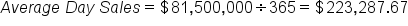The average day sales is \$223,287.67 per day. Now we can calculate the day sales outstanding.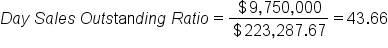This ratio tells us that LMN Company has 43.66 days outstanding, which means that it is taking over 43 days to collect a dollar in sales. This is important in the cash conversion cycle. The LMN Company should look at the credit policy to see if it is too easily granting credit or if the collection policy procedures are too slack. The company should know exactly why the ratio is at 43.66 days.

hint
The accounts receivable can be found on the balance sheet and the total sales can be found on the income statement.

term to know

Day Sales Outstanding Ratio
A ratio that measures the company's average collection period.
1c. Fixed Assets Turnover Ratio
The fixed assets turnover ratio is a ratio that measures sales compared to the value of these fixed assets. This ratio indicates how well the business is using its fixed assets to generate sales. Generally, the higher the ratio, the better. A high ratio indicates that the business has less money tied up in fixed assets for each unit of currency of sales revenue.

This ratio is calculated by dividing the total sales by the average fixed assets.

formula
Fixed Assets Turnover Ratio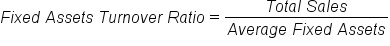term to know

Fixed Assets Turnover Ratio
A ratio that measures sales compared to the value of these fixed assets.
1d. Total Assets Turnover Ratio
The total assets turnover ratio is a ratio that measures the efficiency of a company’s use of its assets in generating sales revenue. Companies with low profit margins tend to have high asset turnover, while those with high profit margins tend to have low asset turnover.

EXAMPLE

Companies in the retail industry tend to have a very high turnover ratio mainly due to cutthroat competitive pricing.

This ratio is calculated by dividing the total sales by the average total assets.

formula
Total Assets Turnover Ratio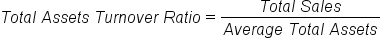term to know

Total Assets Turnover Ratio
A ratio that measures the efficiency of a company's use of its assets in generating sales revenue.
summary
In this lesson, we learned about the different types of asset management ratios. The inventory turnover ratio is a ratio that measures the number of times inventory is sold or used in a time period. The day sales outstanding ratio measures the company’s average collection period. It is a measure of their accounts receivable and how long it takes a company to collect the money for purchases that have been made. The fixed assets turnover ratio is a ratio that measures sales compared to the value of these fixed assets. Finally, the total assets turnover ratio is a ratio that measures the efficiency of a company’s use of its assets in generating sales revenue.

Best of luck in your learning!

Source: THIS CONTENT HAS BEEN ADAPTED FROM LUMEN LEARNING'S “ASSET MANAGEMENT RATIOS” TUTORIAL.

Terms to Know
Days Sales Outstanding Ratio

A ratio that measures the company's average collection period.

Fixed Assets Turnover Ratio

A ratio that measures sales compared to the value of these fixed assets.

Holding Cost

Money spent to keep and maintain a stock of goods in storage.

Inventory Turnover Ratio

A ratio that measures the number of times inventory is sold or used in a time period.

Total Assets Turnover Ratio

A ratio that measures the efficiency of a company's use of its assets in generating sales revenue.

Formulas to Know
Day Sales Outstanding RatioFixed Assets Turnover RatioInventory Turnover RatioTotal Assets Turnover RatioRating